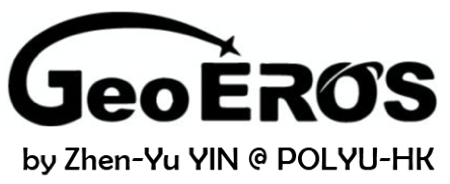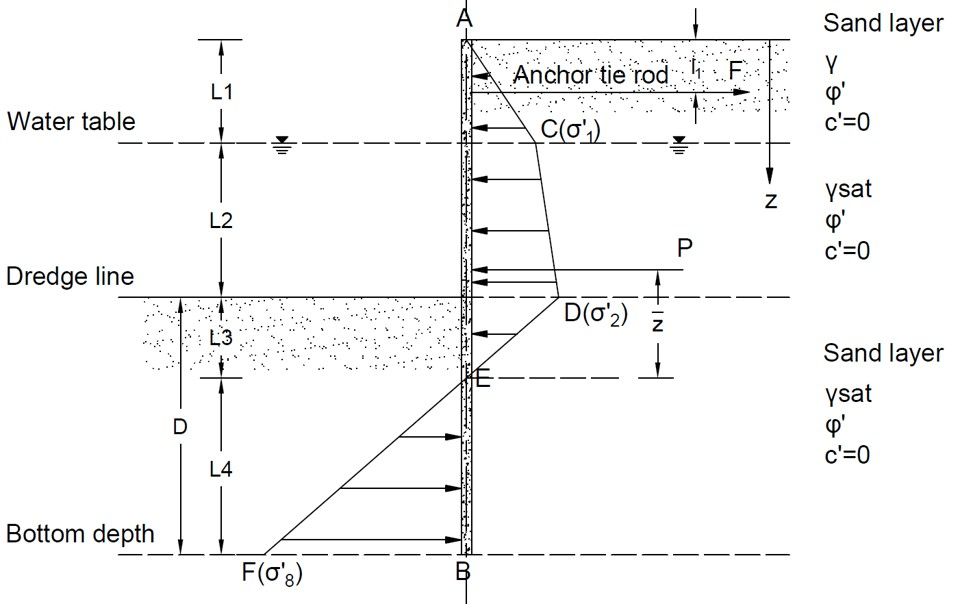# Anchor Sheet Pile Wall Tool (Sand)## Geometry of anchor sheet piling penetrating sandy soils## Tips

• This tool is for analyzing the anchor sheet piling penetrating sandy soils, as shown in the figure above.
• A homogenous soil layer with groundwater is considered. The cohesion of sand layer is assumed to be zero.
• The tie rod of anchor connecting the sheet pile is located above the groundwater table, at a depth of l1.
• The Rankine earth pressure theory is used.
• The unit weight of water is 9.8 kN/m3.
• No surcharge is considered in this case.
• All the input parameters should be positive.
• For "nan", "0" or "inf" displayed in Results, please check your input parameters.

L1 (m)
L2 (m)
l1 (m)
γ (kN/m3)
γsat (kN/m3)
φ′ (°)
σall (MN/m2)

## Output Results

• Magnitude of pressure diagram
σ′1 (kN/m2) =
σ′2 (kN/m2) =
σ′8 (kN/m2) =
• Length of pressure diagram
L3 (m) =
L4 (m) =
• Theoretical embedment depth
Dtheory (m) =
• Center point for pressure of ACDE
z (m) =
• Anchor force per unit length
Fanchor (kN/m) =
• Point for zero shear
z (m) =
• Magnitude of maximum moment
Mmax (kN*m/m) =
• Minimum section modulus
S (10-3m3/m) =